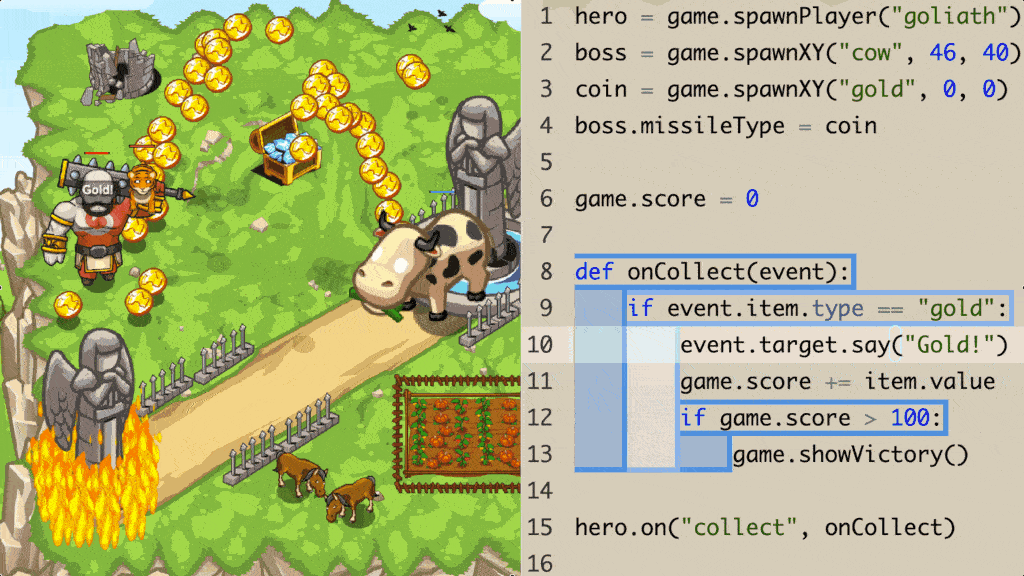# Pre-Python##### Who is this course for
Beginner Students: This course is for students with no previous Computer Science experience.
This course typically takes 3-6 months to complete. This depends on the student's experience and how fast they can master the concepts and the knowledge. Our instructors move at the pace of the student, it may take extra time for some students to reinforce what they have learned.
##### Curriculum
###### Chapter 1: Algorithma step-by-step set of instructions for completing a task. When algorithms are written using code, computers can follow them.
###### Chapter 2: Basic Syntaxthe rules for correct spelling, grammar, and punctuation in a programming language. The syntax must be exactly right for code to execute properly.
###### Chapter 3: Objecta character or thing that can perform actions. Objects are the building blocks of Python. They are things or characters that can perform actions. Your hero is an object. It can perform the moving actions.
###### Chapter 4: Methodan action performed by an object.
###### Chapter 5: Argumentextra information passed into a method to modify what the method does. In Python, arguments are represented by code that is inside the parentheses after a method.
###### Chapter 6: Stringa type of data that represents text. In Python, strings are represented by text inside quotes. In this course, strings are used to identify objects for the hero to attack.
###### Chapter 7: Loopsa programming structure used to repeat actions without the player needing to write the same lines of code over and over.
###### Chapter 8: Variablea symbol that represents data. The value of a variable can be modified over the course of the program. In this course, variables are used to name enemies, then passed along as arguments to the attack method so that the hero can attack the correct enemy.
###### Chapter 9: Functionan action performed by an object. Functions are actions an object can do. moveRight() is a function. Function names are always followed by parentheses.
###### Chapter 10: Conditionalthe building block of modern programming, the conditional. It’s named as such because of its ability to check the conditions at the moment and perform different actions depending on the expression. The player is no longer able to assume there will be an enemy to attack, or if there is a gem to grab. Now, they need to check whether it exists, check if their abilities are ready, and check if an enemy is close enough to attack.
###### Chapter 11: Eventan object representing something that happened. Students can write code to respond to events: when this type of event happens, run this function. This is called event handling, and it's a very useful programming pattern and an alternative to an infinite while-loop.

### You Might Also Be Interested In Our Elective Courses##### Introduction to Number Theory
AGES 10 - 15

This course is designed to build the basics of a complicated mathematical field. It is designed to be a complete introduction to the fundamental concepts of number theory for students in grades 7-10.

The class covers topics such as primes and composites; multiples, divisors, and divisibility; prime factorization and its uses; modular arithmetic and more.##### Introduction to Geometry
AGES 10 - 15

Students in grades 6-10 will build a solid understanding of more advanced geometry concepts in this course. It is designed to expand on the standard high school geometry curriculum and to challenge students with new concepts and problems.

Topics include congruent and similar triangles, circles, 3D geometry, and introductory trigonometry.##### Introduction to Counting and Probability
AGES 10 - 15

To build on the concepts from the Introduction level course, students will build on their Counting and Probability knowledge.

A thorough introduction for students in grades 7-10 to counting and probability topics such as permutations, combinations, Pascal's triangle, geometric probability, basic combinatorial identities, the Binomial Theorem, Pigeonhole Principle Conditional, Probability Graph Theory and more.